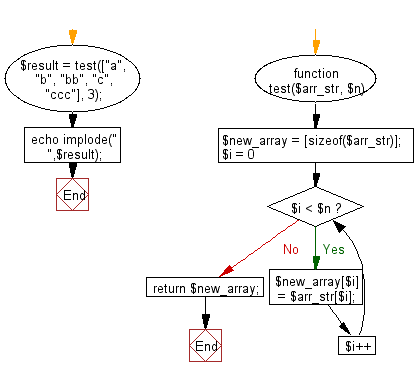﻿ PHP Exercises: Create a new array using the first n strings from a given array of strings - w3resource# PHP Exercises: Create a new array using the first n strings from a given array of strings

## PHP Basic Algorithm: Exercise-134 with Solution

Write a PHP program to create a new array using the first n strings from a given array of strings. (n>=1 and <=length of the array).

Sample Solution:

PHP Code :

``````<?php
function test(\$arr_str, \$n)
{
\$new_array = [sizeof(\$arr_str)];

for (\$i = 0; \$i < \$n; \$i++)
{
\$new_array[\$i] = \$arr_str[\$i];
}

return \$new_array;
}

\$result = test(["a", "b", "bb", "c", "ccc"], 3);
echo implode(" ",\$result);
``````

Sample Output:

```a b bb
```

Flowchart:PHP Code Editor:

What is the difficulty level of this exercise?

﻿

## PHP: Tips of the Day

\$_REQUEST: This SuperGlobal Variable is used to collect data submitted by a HTML Form

Example:

This code save in a php file and run in the browser.

```<!DOCTYPE html>
<html>
<body>

<form method="post" action="<?php echo \$_SERVER['PHP_SELF'];?>">
NAME: <input type="text" name="user">
<button type="submit">SUBMIT</button>
</form>
<?php
if (\$_SERVER["REQUEST_METHOD"] == "POST") {
\$name = htmlspecialchars(\$_REQUEST['user']);
if(empty(\$name)){
echo "Name is empty";
} else {
echo \$name;
}
}
?>
</body>
</html>
```

Output:

```Owen
```